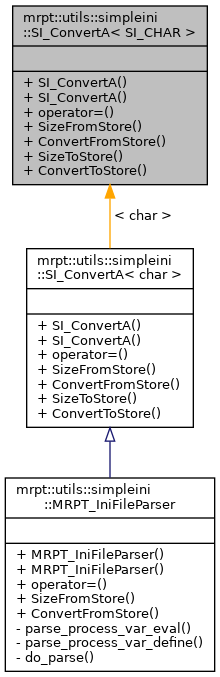Main MRPT website > C++ reference for MRPT 1.5.6
mrpt::utils::simpleini::SI_ConvertA< SI_CHAR > Class Template Reference

Detailed Description

template<class SI_CHAR> class mrpt::utils::simpleini::SI_ConvertA< SI_CHAR >

Null conversion class for MBCS/UTF-8 to char (or equivalent).

Definition at line 1950 of file SimpleIni.h.

`#include <utils/simpleini/SimpleIni.h>`

Inheritance diagram for mrpt::utils::simpleini::SI_ConvertA< SI_CHAR >:Public Member Functions

SI_ConvertA ()

SI_ConvertA (const SI_ConvertA &rhs)

SI_ConvertAoperator= (const SI_ConvertA &rhs)

virtual size_t SizeFromStore (const char *a_pInputData, size_t a_uInputDataLen)
Calculate the number of SI_CHAR required for converting the input from the storage format. More...

virtual bool ConvertFromStore (const char *a_pInputData, size_t a_uInputDataLen, SI_CHAR *a_pOutputData, size_t a_uOutputDataSize)
Convert the input string from the storage format to SI_CHAR. More...

size_t SizeToStore (const SI_CHAR *a_pInputData)
Calculate the number of char required by the storage format of this data. More...

bool ConvertToStore (const SI_CHAR *a_pInputData, char *a_pOutputData, size_t a_uOutputDataSize)
Convert the input string to the storage format of this data. More...

Constructor & Destructor Documentation

template<class SI_CHAR>
 mrpt::utils::simpleini::SI_ConvertA< SI_CHAR >::SI_ConvertA ( )
inline

Definition at line 1952 of file SimpleIni.h.

template<class SI_CHAR>
 mrpt::utils::simpleini::SI_ConvertA< SI_CHAR >::SI_ConvertA ( const SI_ConvertA< SI_CHAR > & rhs )
inline

Definition at line 1955 of file SimpleIni.h.

Member Function Documentation

template<class SI_CHAR>
 virtual bool mrpt::utils::simpleini::SI_ConvertA< SI_CHAR >::ConvertFromStore ( const char * a_pInputData, size_t a_uInputDataLen, SI_CHAR * a_pOutputData, size_t a_uOutputDataSize )
inlinevirtual

Convert the input string from the storage format to SI_CHAR.

The storage format is always UTF-8 or MBCS.

Parameters
 a_pInputData Data in storage format to be converted to SI_CHAR. a_uInputDataLen Length of storage format data in bytes. This must be the actual length of the data, including NULL byte if NULL terminated string is required. a_pOutputData Pointer to the output buffer to received the converted data. a_uOutputDataSize Size of the output buffer in SI_CHAR.
Returns
true if all of the input data was successfully converted.

Reimplemented in mrpt::utils::simpleini::MRPT_IniFileParser.

Definition at line 1997 of file SimpleIni.h.

template<class SI_CHAR>
 bool mrpt::utils::simpleini::SI_ConvertA< SI_CHAR >::ConvertToStore ( const SI_CHAR * a_pInputData, char * a_pOutputData, size_t a_uOutputDataSize )
inline

Convert the input string to the storage format of this data.

The storage format is always UTF-8 or MBCS.

Parameters
 a_pInputData NULL terminated source string to convert. All of the data will be converted including the terminating NULL character. a_pOutputData Pointer to the buffer to receive the converted string. a_uOutputDataSize Size of the output buffer in char.
Returns
true if all of the input data, including the terminating NULL character was successfully converted.

Definition at line 2041 of file SimpleIni.h.

template<class SI_CHAR>
 SI_ConvertA& mrpt::utils::simpleini::SI_ConvertA< SI_CHAR >::operator= ( const SI_ConvertA< SI_CHAR > & rhs )
inline

Definition at line 1956 of file SimpleIni.h.

template<class SI_CHAR>
 virtual size_t mrpt::utils::simpleini::SI_ConvertA< SI_CHAR >::SizeFromStore ( const char * a_pInputData, size_t a_uInputDataLen )
inlinevirtual

Calculate the number of SI_CHAR required for converting the input from the storage format.

The storage format is always UTF-8 or MBCS.

Parameters
 a_pInputData Data in storage format to be converted to SI_CHAR. a_uInputDataLen Length of storage format data in bytes. This must be the actual length of the data, including NULL byte if NULL terminated string is required.
Returns
Number of SI_CHAR required by the string when converted. If there are embedded NULL bytes in the input data, only the string up and not including the NULL byte will be converted.
-1 cast to size_t on a conversion error.

Reimplemented in mrpt::utils::simpleini::MRPT_IniFileParser.

Definition at line 1973 of file SimpleIni.h.

template<class SI_CHAR>
 size_t mrpt::utils::simpleini::SI_ConvertA< SI_CHAR >::SizeToStore ( const SI_CHAR * a_pInputData )
inline

Calculate the number of char required by the storage format of this data.

The storage format is always UTF-8 or MBCS.

Parameters
 a_pInputData NULL terminated string to calculate the number of bytes required to be converted to storage format.
Returns
Number of bytes required by the string when converted to storage format. This size always includes space for the terminating NULL character.
-1 cast to size_t on a conversion error.

Definition at line 2021 of file SimpleIni.h.

 Page generated by Doxygen 1.8.6 for MRPT 1.5.6 Git: 4c65e84 Tue Apr 24 08:18:17 2018 +0200 at mar abr 24 08:26:17 CEST 2018